Next: 5. Real Numbers Up: 4. The Complexification of Previous: 4.1 Construction of .   Index

# 4.2 Complex Conjugate.

4.21   Definition (Complex conjugate.) Let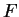be a field in which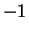is not a square. Let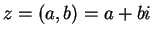be an element of. We define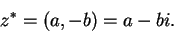is called the conjugate of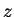.

The following remark will be needed somewhere in the proof of the next exercise.

4.22   Remark. Ifis a field in whichis not a square, then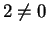in, since4.23   Exercise. A Letbe a field in whichis not a square. Let. Show that
a).
b)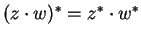.
c)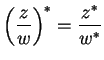if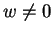.
d)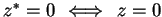.
e)
If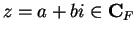, then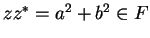. Ifthen.
f)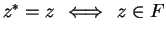.
g).

4.24   Example. The results of the previous exercise provide a way to write expressions of the form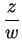in the form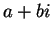. Write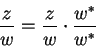and calculate away. For example, in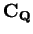, we have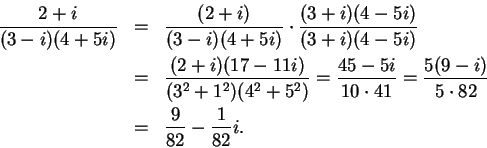4.25   Exercise. A Write each of the following elements ofin the formwhere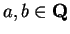.
a)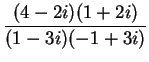b)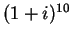4.26   Note. The first appearance of complex numbers is in Ars Magna (1545) by Girolamo Cardano (1501-1576).

If it should be said, Divide 10 into two parts the product of which is 30 or 40, it is clear that this case is impossible. Nevertheless, we will work thus:[16, page 219].
He then proceeds to calculate that the parts are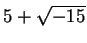and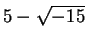, and says
Putting aside the mental tortures involved, multiplyby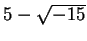making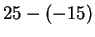which is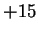. Hence this product is 40. So progresses arithmetic subtlety the end of which, as is said, is as refined as it is useless [16, page 219-220].

Around 1770, Euler wrote

144. All such expressions as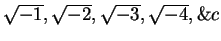are consequently impossible, or imaginary numbers, since they represent roots of negative quantities; and of such numbers we may truly assert that they are neither nothing nor greater than nothing, nor less than nothing; which necessarily constitutes them imaginary, or impossible.

145. But notwithstanding this, these numbers present themselves to the mind; they exist in our imagination, and we still have a sufficient idea of them; since we know that byis meant a number which, multiplied by itself, produces; for this reason also, nothing prevents us from making use of these imaginary numbers, and employing them in calculation. [20, p 43]

The use of the letterto represent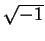was introduced by Euler in 1777.[15, vol 2, p 128] Both Maple and Mathematica use I to denote.

The first attempts to justify'' the complex numbers appear around 1800. The early descriptions were geometrical rather than algebraic. The algebraic construction ofused in these notes follows the ideas described by William Hamilton circa 1835 [25, page 83].

You will often find the complex conjugate ofdenoted by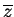instead of. The notion of complex conjugate seems to be due to Cauchy[45, page 26], who calledand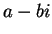conjugates of each other.Next: 5. Real Numbers Up: 4. The Complexification of Previous: 4.1 Construction of .   Index